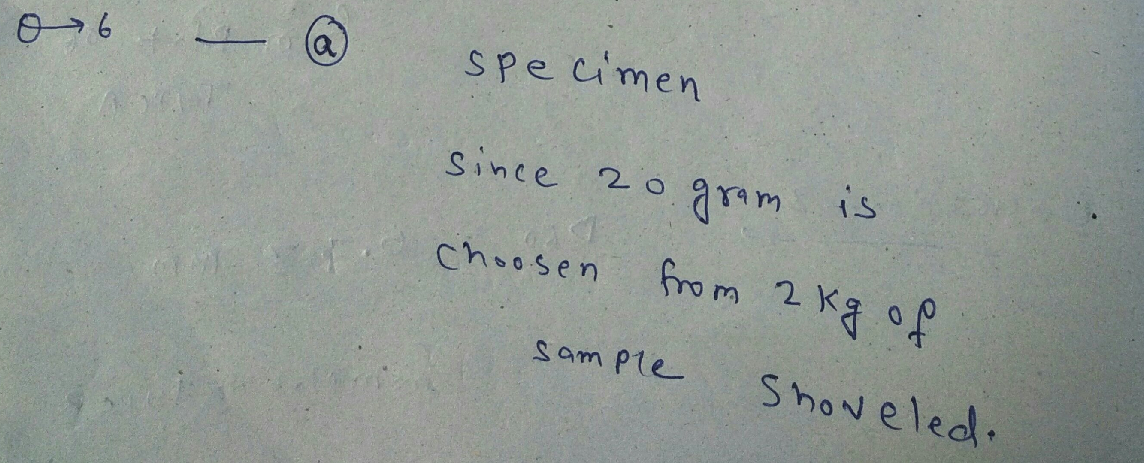Question

Geotechnical / Foundation Engineering

Tom Zink went out to Fresno, CA and shoveled 2 kg of sand into a bucket and brought the sand to his lab for testing. He then took 20 grams of sand from the bucket and compacted the 20 grams in a die. He then loaded the compacted sand with a piston using a load frame. During loading, the 20 gram sand pack was called

a.a specimen b.a sample C. gravel d. clayVerified### Question 45698Geotechnical / Foundation Engineering

9. What would the FS for the potential failure surface shown above be if the soil's undrained shear strength were 50 kPa (instead of 100 kPa)? Answer: FS = 0.98

### Question 45697Geotechnical / Foundation Engineering

8., Find the factor of safety for the failure surface shown, below using the Swedish Slip Circle method. The scale is in meters (use a ruler). The center of the circle is denoted by the •. Answer: FS 1.9550

### Question 43569Geotechnical / Foundation Engineering

50 tons as shown in Figure P8.11. Determine the averagestress increase beneath the center of the foundation in theA square foundation, 5 ft wide, carries a net column load ofAsquareclay layer:
a. Using Eq. (8.25),
b. Using Eqs. (8.26) and (8.10), and
c. Using Eqs. (8.26) and (8.15).

### Question 43568Geotechnical / Foundation Engineering

Three continuous foundations are shown in Figure P6.15.For each of them, what values would you use for their eccentricity and inclination in the bearing capacity calculations?

### Question 43567Geotechnical / Foundation Engineering

A 2 m x 3 m spread footing placed at a depth of2 m carries a vertical load of 3000 kN and a moment of300 kN m, as shown in Figure P6.14. Determine the factor of safety using Meyerhof's effective area method.

### Question 43566Geotechnical / Foundation Engineering

For an eccentrically loaded continuous foundation on sand,given B= 108 m Df=0.9 m e/B =0.12( one way eccentricity Y=16KN/m3 and theta =35 using the reduction factor method estimate load per unit length of the foundation

### Question 43565Geotechnical / Foundation Engineering

Repeat Problem 6.11 using Prakash and Saran's method.

### Question 43564Geotechnical / Foundation Engineering

An eccentrically loaded foundation is shown in Figure P6.11.Use FS of 4 and determine the maximum allowable load that the foundation çan carry, Use Meyerhof's effective area method.

### Question 43563Geotechnical / Foundation Engineering

For the design of a shallow foundation, given the following:
\phi^{\prime}=20^{\circ}
c^{\prime}=72 \mathrm{kN} / \mathrm{m}^{2}
\text { Unit weight, } \gamma=17 \mathrm{kN} / \mathrm{m}^{3}
Modulus of elasticity, E, = 1400 kN/m-%3D
\text { Poisson's ratio, } \mu_{s}=0.35
Foundation:L= 2 m
B = 1 m
D, = 1 m
Calculate the ultimate bearing capacity. Use Eq. (6.42).

### Question 43562Geotechnical / Foundation Engineering

A column foundation (Figure P6.9) is 3 m X 2 m in plan.Given: D; = 1.5 m, 6' = 25°, c' = 70 kN/m?. UsingEq. (6.28) and FS[see Eq. (6.24)] the foundation could carry.= 3, determine the net allowable load

### Submit query

Getting answers to your urgent problems is simple. Submit your query in the given box and get answers Instantly.

### Submit a new QuerySuccess

Assignment is successfully created